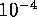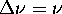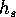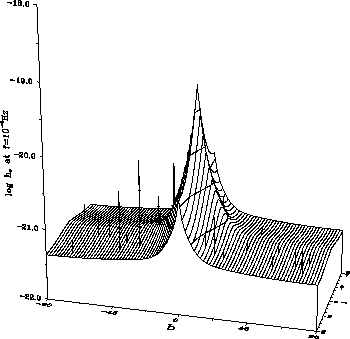Next: Evolution of Supernova Explosion Up: Gravitational Waves from Coalescing Previous: Gravitational Waves from Coalescing

### Gravitational wave background from nearby galaxies

The stochastic binary background produced by nearby galaxies from Tully's catalog at the frequencyĀHz is shown in FigureĀ49 in terms of average strain metric amplitude per square degree in a given direction (l,b) on the sky:where the sum is calculated over all sources in the given square degree of the sky; we assumed.

The lines of constanton the sky (so-called isograwes)Ā are plotted in FigureĀ50. As we expected (see Section 15.9), only the closest and most massive galaxies (such as SMC, LMC, M31, M82, M87) can be clearly discerned by a GW detector with angular resolution of 1against the galactic binary GW stochastic background.

ĀFigure: Stochastic GWB produced by binaries from Tully's catalog against the GWB from our Galaxy at the frequencyĀHz, in terms of mean strain metric amplitude per square degree in a given direction (Lipunov et al., 1995a).Ā

ĀFigure 50: The ``isograwes'' from Figure 49 projected on to (l,b)-plane (Lipunov et al., 1995a).Ā

Mike E. Prokhorov
Sat Feb 22 18:38:13 MSK 1997